Latest Banking jobs   »

# Reasoning Quiz For RBI Grade B Phase 1 2023-18th March

Directions (1-5): Read the following information carefully and answer the given questions.
Eight persons A, B, C, D, E, F, G and H live on different floors of the same building having eight floors (the ground floor is numbered as the first floor while the topmost floor is numbered as the eighth floor). They were born on the same day of the same month but in different years. All their ages are to be considered from the base year i.e., 2018.
G is 42 years old and lives on an odd numbered floor below the 5th floor. B is 20years younger than the one who lives on 2nd floor. Only three persons live between G and the one who was born in 1984. F lives on 4th floor and he is 13 years old. A lives on even numbered floor. There are three floors in between C and D who lives on an even numbered floor. H is 34 years old and lives on an odd numbered floor. The one who is 28 years old lives immediate above the floor on which E lives. The one who lives on ground floor is 5 years older than the one who lives on 4th floor. D was born in 1996. B lives above the floor on which C lives. One person was born in 1962.

Q1. How many people live between B and the one who was born in 1990?
(a) Four
(b) Three
(c) Two
(d) One
(e) More than Four

Q2. Who among the following lives on top most floor?
(a) The one who was born in 1962
(b) D
(c) E
(d) H
(e) C

Q3. Who among the following lives on 5th floor?
(a) The one who is 8 years old
(b) R
(c) The one who was born in 1984
(d) C
(e) E

Q4. Who among the following lives just below the one who was born in 1976?
(a) D
(b) B
(c) C
(d) A
(e) F

Q5. Which of the following combinations is true?
(a) Fifth floor-F
(b) Second floor-E
(c) Sixth floor-G
(d) Eighth floor -A
(e) Third floor-H

Directions (6-10): In each of the questions given below, a group of digits is given followed by four combinations of letters/ symbols numbered. You have to find out which of the four combinations correctly represents the group of digits based on the letter/ symbol codes and the conditions given below. If none of the four combinations represents the group of digits correctly, give (e) i.e. ‘None of these’ as the answer.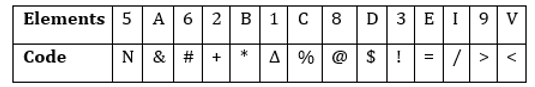Condition for coding the group elements:
(i). If the first number is even and last letter is vowel, then the codes for the first and the last digits are to be interchanged.
(ii). If the first as well as the last letter is consonant, then both are to be coded by the code for the fourth letter.
(iii). If the first as well as the last number is odd, then both are to be coded by the code for the last number.
(iv). If the first number is odd and last letter is consonant, then both are to be coded by the code for the first number.

Q6. 5A8DCB9
(a) >&@\$*%>
(b) N&@\$%*>
(c) >&@\$%*N
(d) >&@\$%*>
(e) None of these

Q7. E31VI8D
(a) \$!∆</@\$
(b) =!∆/<@\$
(c) =!∆</@\$
(d) =!∆</@!
(e) None of these

Q8. C9AV32B
(a) %>&<!+<
(b) <>&<!+<
(c) <<&<!+<
(d) %>&<!+*
(e) None of these

Q9. 8A9D1IE
(a) =&\$>∆/@
(b) =&>\$∆=
(c) @&>\$∆=
(d) =&>\$∆/@
(e) None of these

Q10. 3BD8ACV
(a) !*\$@%&!
(b) !*\$@&%!
(c) !*\$*&%!
(d) !*#@&%!
(e) None of these

Directions (11-14): Read the following information carefully and answer the questions given below it.
P% Q means P is sibling of Q
P\$Q means P is parent of Q
P#Q means P is grandparent of Q
P&Q means P is spouse of Q
+P/+Q represents the male members
-P/-Q represents the female members
[Note: consider only given persons in the question]

Q11. If the expression “G\$ D % (-) C &A\$B& (+) E; C # (+) F%J” holds true, then how is A related to F?
(a) Maternal grandfather
(b) Paternal grandmother
(c) Paternal grandfather
(d) Uncle
(e) None of these

Q12. If the expression “G\$ D % (-) C &A\$B& (+) E; C# (+) F%J” holds true, then how many married couples are there in the family?
(a) One
(b) Three
(c) Two
(d) None of these
(e) Four

Q13. If the expression “G\$ D % (-) C &A\$B& (+) E; C# (+) F%J” holds true, then how is A related to G?
(a) Son
(b) Son in law
(c) Brother
(d) None of these
(e) Brother-in-law

Q14.In the given expressions “G\$ D % (-) C &A\$B& (+) E; C# (+) F%J”, if the age of C is 68 and the age of F is 25 then what is the possible age of B?
(a) 23
(b) 70
(c) 40
(d) 20
(e) 75

Directions (15): Study the following information carefully and answer the questions given below:
P%Q means P is sibling of Q
P\$Q means P is parent of Q
P#Q means P is grandchild of Q
P@Q means P is child of Q
P&Q means P is spouse of Q

Q15. If the expression “B\$C@A; D#E&F\$B” hold true, then how C is related to D?
(a) Brother
(b) Sister
(c) Either (a) or (b)
(d) Mother
(e) Father

Solutions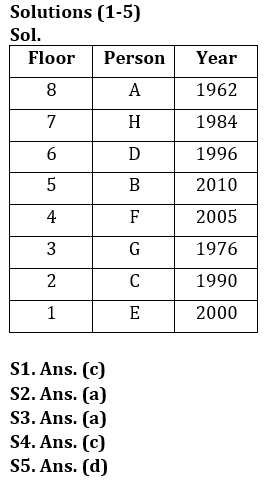Solutions.(6-10):
S6. Ans.(d)
Sol. Condition (iii) applies
S7. Ans.(c)
Sol. None of the conditions are applied.
S8. Ans.(b)
Sol. Condition (ii) applies
S9. Ans.(d)
Sol. Condition (i) Applies.
S10. Ans.(b)
Sol. Condition (iv) Applies.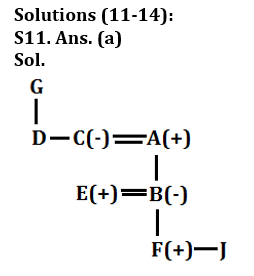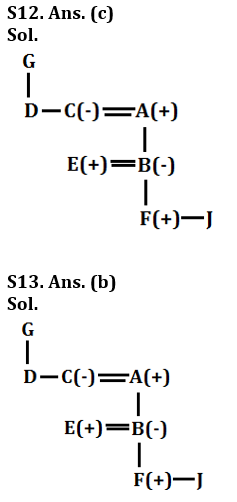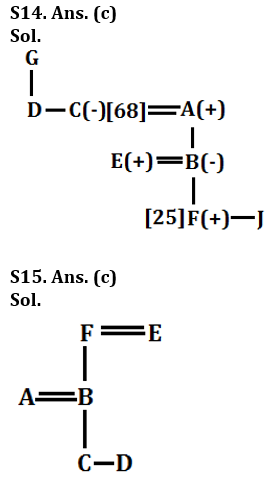## FAQs

### What is the selection process of the Bank Clerk?

There are 4 sections in the RBI Grade B Phase 1 Exam i.e. English Language, General Awareness, Quantitative Aptitude & Reasoning.

#### Congratulations!Union Budget 2023-24: Free PDF